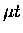Next: Back-decayed energy distribution Up: Effective formation model Previous: Epithermal Collisions

## Comparison with the explicit back decay model

We illustrate the effect of back decay in the following simplified comparison. We consider a case in which abeam of resonant energy collides with a D2 layer of varying thickness, and we wish to describe the fusion yield in the D2 layer as a function of its thickness. We compare the two models (a) using effective formation rates renormalized for the back decay fraction as was used in Ref. [75,27,9,82], and model (b) using the total formation rate and explicit back decay. We consider only two competing processes of elastic scattering and molecular formation, the latter leading to either fusion or back decay, and neglect other possibilities such as scattering followed by molecular formation (muon decay is also neglected).

With these assumptions, the fusion yield as a function of layer thickness in the effective formation model (a) can be expressed as:

 (128)

where x (cm-2) is the thickness multiplied by number density n, and cross sections and rates are related with , v being velocity of thefor a target molecule at rest. With back decay explicitly included (model b), the fusion yield as a function of layer thickness is now written:

 (129)

These expressions can be expanded in series in x:

 Ya (130) Yb (131)

Recalling , both models give the same results to leading order. Therefore, in the limit of a thin layer, model (a) can be justified for describing the fusion yield. However, the next-to-leading order corrections become important at x comparable to the mean interaction length, . In the limit of a thick layer ( ), the fusion yield is simply the coefficient in Eqs. B.4 and B.5. Comparing Eq. B.6 and B.7, it is clear that

 (132)

resulting in an overestimate of fusion yield with model (a).

In our earlier analysis of the time-of-flight fusion experiments, the effective rate (model a) was used, and we observed that the calculated fusion yield was significantly larger than the experimental data. Since our thickness was comparable to the interaction length xint, the discussion given here explains the discrepancy. Detailed Monte Carlo calculations indeed show that the fusion yield is overestimated by nearly 50% in our time-of-flight fusion measurement arrangement, compared to model (b).

It is interesting to note that Eq. B.5 suggests that, within model (b) and given the knowledge of , one can determine both and W by the absolute measurement of the thickness dependence of fusion yield Y(x), i.e., from the curvature of Y(x), and W from Y(x) at the limit of large x(via the coefficient ). Note that it is difficult to disentangle and W in conventional measurements using a homogeneous D/T mixture.Next: Back-decayed energy distribution Up: Effective formation model Previous: Epithermal Collisions